Function Repository Resource:

# NaNQ

Test if a numeric value is an IEEE 754 NaN

Contributed by: Ting Sun
 ResourceFunction["NaNQ"][x] gives True if real number x is an IEEE 754 NaN and False otherwise.

## Details

IEEE 754 NaN values are usually imported as real numbers via LibraryLink or other extenral numeric systems (e.g. NumPy).
Some usages should be converted to Indeterminate prior to further computation.

## Examples

### Basic Examples (1)

Introduce an IEEE 754 NaN value and test it:

 In:=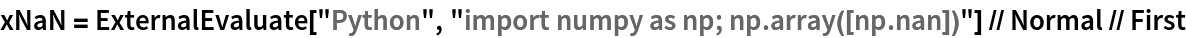Out=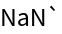In:=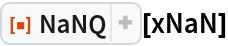Out=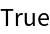### Applications (1)

Detect IEEE 754 NaN values and replace them with Indeterminate for further computation:

 In:=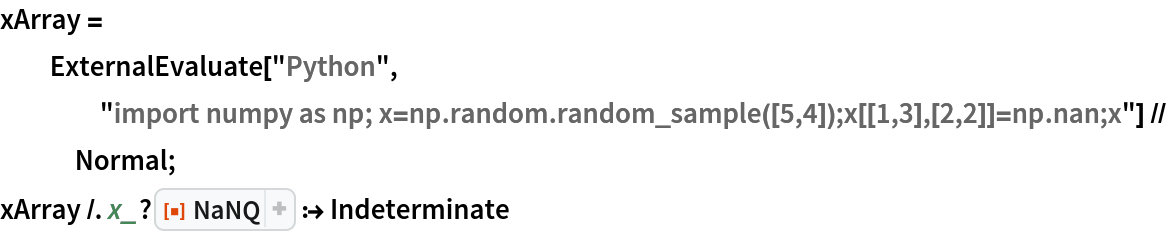Out=### Possible Issues (2)

Direct computation involving NaN values may give unexpected results:

 In:=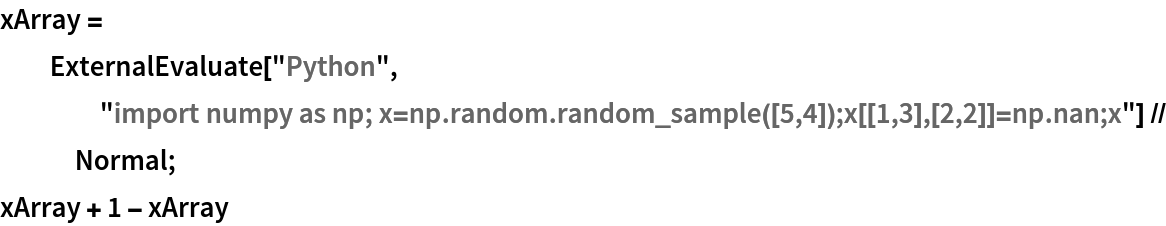Out=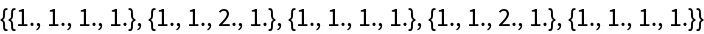NaNs should be replaced by Indeterminate for further computation:

 In:=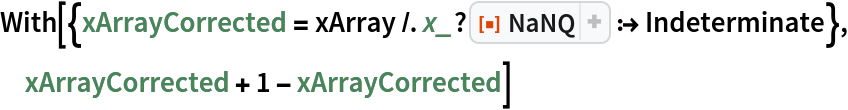Out=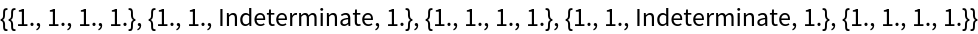Ting Sun

## Version History

• 1.0.0 – 26 April 2021